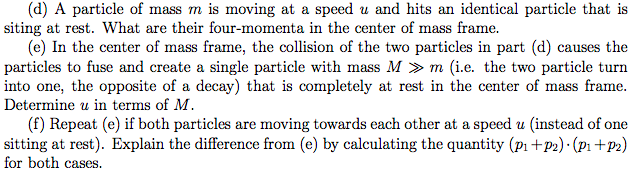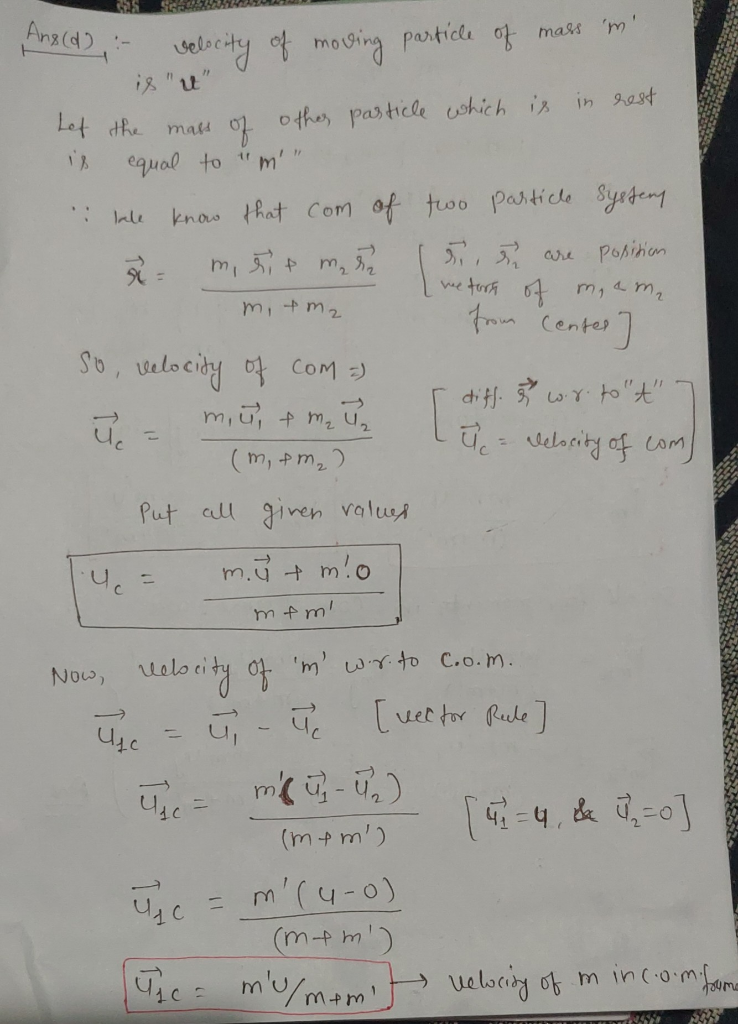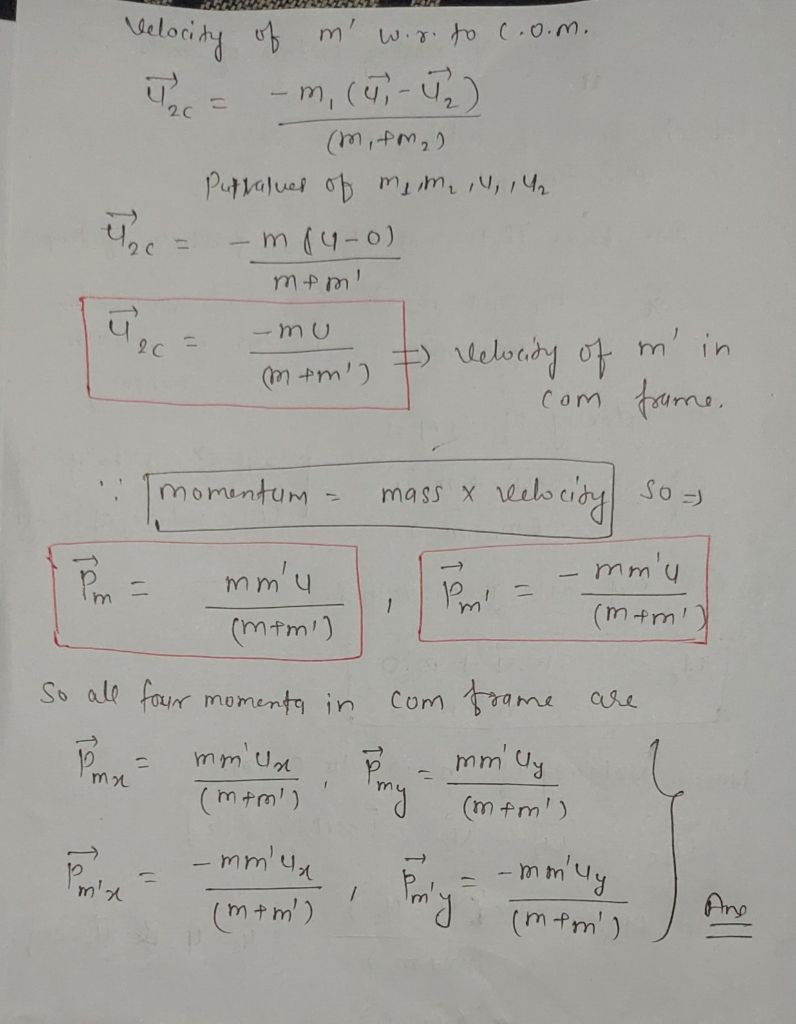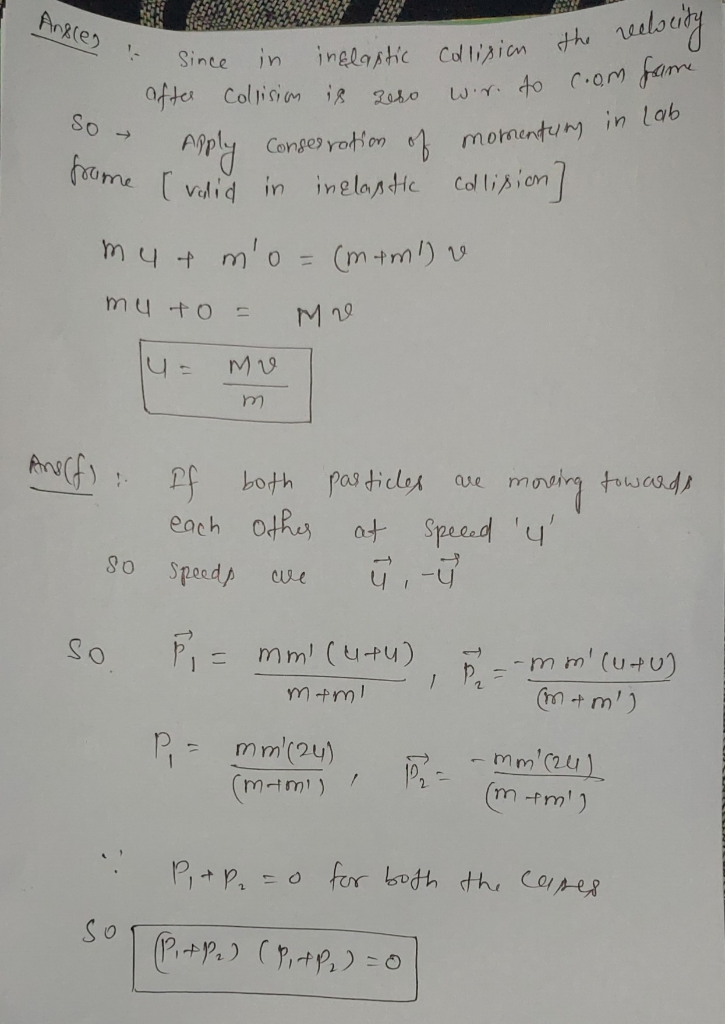# (d) A particle of mass m is moving at a speed u and hits an identical particle that is siting at ...(d) A particle of mass m is moving at a speed u and hits an identical particle that is siting at rest. What are their four-momenta in the center of mass frame (e) In the center of mass frame, the collision of the two particles in part (d) causes the particles to fuse and create a single particle with mass M »m (i.e. the two particle turn into one, the opposite of a decay) that is completely at rest in the center of mass frame. Determine u in terms of M. (f) Repeat (e) if both particles are moving towards each other at a speed u (instead of one sitting at rest). Explain the difference from (e) by calculating the quantity (Pi +P2) (pi+P2) for both cases.##### Add Answer of: (d) A particle of mass m is moving at a speed u and hits an identical particle that is siting at ...
More Homework Help Questions Additional questions in this topic.

• #### In the figure below, two tiny conducting balls of identical mass m and identical charge q hang...

Need Online Homework Help?